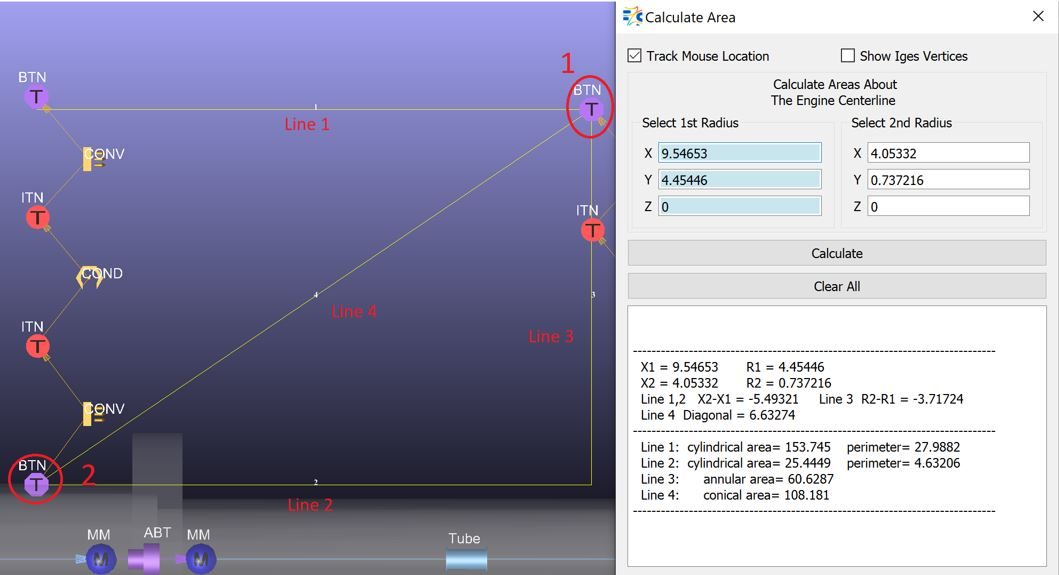# Calculate Area

Calculate Area option allows the user to measure the area between two points. Similar to the Calculate Distance option, there are 3 major way to select points:

1. Track Mouse Location: tracks the mouse and gets the X, Y, Z location at the mouse clicked. The first click will set the X, Y, Z locations of the 1st point and the second click will be for 2nd point.

2. Show Iges Vertices: If there is a loaded CAD model, it is possible to select 2 of these points to measure the distance between them. The Iges vertices can only be seen when this option is enables.

3. Write the X, Y, Z locations of the 1st and 2nd points directly.Calculate: To calculate the area, after setting the locations of the points, the user should click on Calculate.

Clear All: To clear the points’ locations and the calculations.

X1 = 1st Point’s X location

R1 = 1st Point’s Y location

X2 = 2nd Point’s X location

R2 = 2nd Point’s Y location

Line 1: cylindrical area = 2*π*R1*(X2-X1)

Line 2: cylindrical area = 2*π*R2*(X2-X1)

Line 3: annular area = π*(R22-R12)

Line 4: conical area =